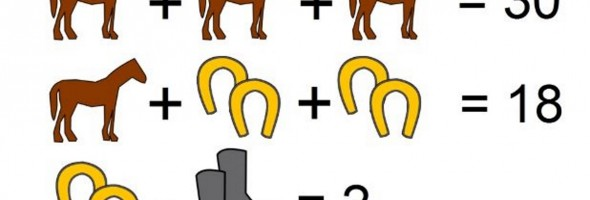## Simple Horseshoe Equation Has Internet Stumped – Can You Solve This Viral Math Problem?Tricky math problems are all the rage these days, especially the seemingly simple ones.

This one is no exception. At first glance, this math problem seems easy enough. It’s just a series of simple equations, each leading to the next. No brainer, right?Well once you learn the answer to this problem, you may have a different opinion.

The beginning of the equation is a no-brainer, almost too easy in fact. If three horses equals 30, then one horse must equal 10!At this point, we can just plug in “10” for the horses and solve from there. So far so good.

Now we can move down to the next line. If we know that one horse equals 10, then we can easily solve for the horseshoes by plugging in “10” for the horse.

This is where the problem starts to get tricky. While it is still simple algebra, there is one key piece most people overlook.Did you get 4? If so, think again. Instead of x+x=8, think 2x+2x+8.

Now that you know what to look for, are you one of the few who will solve the rest of the problem correctly?

Here it is one more time, plus a little hint.